﻿ Flux Particle Theory a Complete Theory of EverythingChange Font Size: A A A Properties of Energy & Matter... Common knowledge in the 21st century By James Clifford Cranwell 2/17/98
 Dimensionality | Flux | Gravity | Atomic Structure | Relativity | Big Bang | Misc. |Elliptical Orbit Gravitational Engine

 Everything in the Universe is made from one type of particle. All workings of the Universe are result from said particle.Abstract:

"Flux Particle Theory... How to make the most universe with the least amount of material"

Every first year Chemistry student learns of the proton to electron orbital ratio (how an atom is mostly empty space). But if you asked the same student what a three dimensional object is they would undoubtedly say a solid. To me this is just flat out wrong (nothing is solid).
If we look back in history, there was a point in time when everyone thought the Earth was the center of the universe and the Sun revolved around it. Even the educated people of the day wouldn't have had any doubt to the validity of this concept, based on the fact that you can actually see the Sun rise and set (supposedly orbit) around the Earth on any given day.
Extremely accurate measurements of the Suns orbit could have also been taken. Leading the whole scientific community to believe they have proved something to ten or twelve decimal places, ...not only falsely confirming the theory, but also inadvertently giving a fundamentally incorrect foundation for others to build upon, that's an extremely important point... even though all the egg-heads concur on something... they can see it, measure it, derive a math formula about it... they are all backwards and thereby wrong.
Therefore, even though everyone thinks something works in a certain way and can actually see it with their own two eyes, there is still an allowance for something happening in a completely different manner, and there is always an easier explanation or a different way to explain something. There can even be a completely new math based on particle lengths, it doesn't mean everything else is incorrect ... it just means this can also be correct and deserves consideration.

Flux theory is a complete theory of everything ... including Gravity, The four forces, and a completely new way to understand the way dimensionality actually works. It is based solely on irrefutable logic. And although it is easily understandable by anyone, it requires an open mind and a completely new way of thinking.

Flux Theory explains (or you will automatically understand) Gravity, Mass, Particle shapes, The Double Slit Experiment, The Four Forces, The Fifth Force, Fringe Patterns, Dark Matter, Missing Matter in the Universe, Binding energy, Alpha Beta Gamma Radiation, Atomic Structures, Induced Fields, Interference, Photo Electric Effect, Polarization of Light, Wave / Particle Duality, Zeeman Effect, Zero-point Energy, Why Superstring theories work mathematically in ten dimensions, Previously unknown effects, Constants and Theories of others (if they were on the right track). It makes everything fall into place.
... A great scientist once said the Grand Unified Field might be a geometric problem and that's exactly what this solution is.

 Properties of Energy & Matter | Part 1 Dimensionality |

 by James Clifford Cranwell 3/27/98 Part I ... Spatial DimensionalityContrary to popular belief... nothing is even remotely solid. At the sub atomic level it is well known the nucleus radius to electron orbital ratio is one hundred thousandth.
That makes the volumetric or spatial difference one quadrillionth = 10-15 = ( .000 000 000 000 001 ) . This ratio is approximately the same size as a spherical dot above the  letter " i " (the proton) on the fifty - yard line in a football stadium (the orbital) everything else is empty space. So if we think of or visualize a huge sphere the size of a stadium (a small moon for instance) in reality the amount of actual continuous mass (just nuclei)
 (4/3)Pi 13 ---------------- (4/3)Pi 100,0003 = 1 --------------------- 1,000,000,000,000,000 = one  quadrillionth
is equivalent to a solid dot above the " i " made of only protons cut up into one quadrillion times 1,000 billion billion pieces and evenly dispersed (that's one quadrillion "i" dots to fill the moon sized sphere (ignoring sphere packing) then 1,000 billion billion atomic radii to fill the "i" dot). That's why neutrinos can zip right through anything completely unfettered and why the moon is only there when someone is looking at it... if we couldn't see vibrations of electron energy, the moon would be completely unnoticed.

Quantum Math
When we differentiate, we get the instantaneous change in whatever equation or shape we consider. It's easy to visualize because the starting equation or figure, for instance a 3-dimensional cube ( y = x3 ), gets lowered down a degree in power or dimension. i.e. ( y' = 3x2) this can be equated to three planar two dimensional sides of the cube or the instantaneous change needed to increase the length, width and height (volume) of the cube, so, this means to instantaneously increase volume, tack on area to half the outside surface. Taking this one step further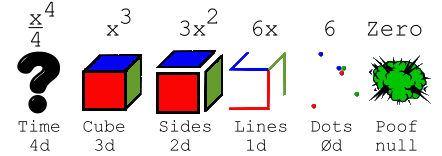we arrive at ( y'' = 6x ) this is simply six one dimensional lines or the instantaneous change needed to increase the length and width (area) of the three planes, every plane needs two lines (length and width) to increase its area, three planes times two lines equals six lines total.
Now it gets even easier ... six points ( y''' = 6 ) are the instantaneous change needed to increase six lines in length (notice the six points are zero-dimensional). Now we arrive at ( y (4 ) = 0 ) this is the instantaneous change needed to take the six points out of existence.
A big problem occurs when we try to integrate something cubic ( y = x3 ) into the fourth dimension, in this case ( integral y ), we have an exact mathematical representation of it ( x 4 /4 ) and if x = 1 we know this is equal to 1/4's worth of fourth dimensional volume (tesserarea?) but, what shape is it? Is it a snapshot in time? Is it an hypercube? Is it a mysterious visitor from the fourth dimension?

The cube isn't solid...Remember, nothing is even remotely solid, so you will rack your brain trying to visualize the integration of a solid or in this case an actual misconception. The mysterious fourth dimensional shape is a tetrahedral axes shaped particle group of higher density. Any one dimensional object is a line. Any two dimensional object is a plane, but that's a slice of a supposed cube, and can't be thought of as for instance a sheet of paper because, if we integrate enough of them into a stack, we have a solid cube of paper and by now we know nothing is solid.
The cube can't be solid. So that form of thinkingis simply wrong (note: The one dimensional line would also have to be in segments, and although we can actually integrate lines into a plane ... the lines in this case are never arranged parallel so they won't form a continuous plane that could then be integrated into a stack)...

The cube isn't solid but since it is there, it must be made of something. If we call the basic unit of whatever the cube is comprised of a particle. The particle must be capable of conveying information, for instance electro - magnetic vibrations. And since there are different frequencies and / or strengths of vibrations with multiple simultaneous combinations, a zero-dimensional single point particle would be incapable of achieving this. It can spin or move or remain at rest but there is no chance of Simultaneity or vibrations. The next possible alternative is the one dimensional line or string (any intrinsic universal characteristic will always be the simplest and at the same time most efficient option).
The string seems to be the shape of choice in this case. On a musical instrument, a violin for instance, the string can convey a multitude of vibrations, tones and harmonics. This means there can be a lot of simultaneous information transmitted along a one dimensional string. There is no need to attempt theoretical construction of a particle made of two dimensional planes because ... if we integrate enough of them into a stack...

Particle Integration :

 x4/4 = 1/4 x3 = 1 3x2 = 3 6x = 6
...So, the basic building blocks of particle construction must be line segments or strings. Their arrangement makes all the difference... The basic unit of the x 3 variety is the X-Y-Z axes shaped particle, this is three strings joined at their centers. If in this example x = 1, then the three axis will be 1/3 in length (X, Y and Z are 1/3 long) with a total length of one. All widths are infinitesimal. When we differentiate x3 we get 3x2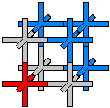this is 3 plus signs or XY axes shaped particles with a total length of 3, that makes 6 axis with 1/2 length... this is also the exact amount of length needed to make three cubic particles... the XYZ's with 1/3 axis length, and the correct amount to add one particle either cubic XYZ or quadratic XY to every axis of the original differentiated particle giving the XYZ an instantaneous change.So, working this in reverse we see that as we integrate into a higher power it changes the shape by adding an axis and it shrinks in size giving it a greater density. So the fourth dimensional object is composed of tetrahedral axes shapes, in this example 1/4 total length f (1) = x4 /4 = 1/4 with 1/16 length axis ( one fourth of 1/4 total ), it gets small very rapidly. More particles are needed to fill any volume because of the shorter lengths and tighter pack, ergo higher density. Now it is easy to see... in a field of 2-D ( XY ) particles we can only traverse horizontally and vertically. When we bump this up one dimension into 3-D we also have the toward and away axis, alas we still can't move on a diagonal, for that is reserved for the higher dimensions.
Now it's a piece of cake to see how any length, for instance 1/(10 * √26 - 1)c, divided up correctly can be a direct representation of a particle(s).

Spatial dimension is directions.

An Abbott Flatlander from Flatland living on a two dimensional plane would actually be living on an infinity of dimensionsif he can turn or move through every angle or vector direction on the supposed plane. The way this actually works is by using axial directions as dimension. An actual working two dimensional model of space would be an infinite array of 2-D axis shaped particles arranged in a plane with the negative or expansive force vibrating through their continuum (matrix). In this 2-D model light is coerced into traveling in straight lines in only two directions (This model can also warp or flex, forcing the curvature of light). If you took enough 2-D particles and curved and connected them into a spherical surface shape, it would be misinterpreted as 3-D.
The actual 2-D electron is pulling this same prank by orbiting spherically and mimicking a 3-D solid, nothing is actually 3-D and/or solid. Now that we know the basic workings of particles at the quantum level and we know vibrations occur in every possible direction, a 3-dimensional particle will not sufficiently transmit vibrations along a diagonal, so, 3-D doesn't work. The particle capable of angular conveyance must be of higher dimension and have the most efficient shape to pack space. It turns out to be a particle with 10-dimensions or ten axes. In the ten dimensional dodecahedral matrix, light is actually forced into zigzags.
This axial concept allows for an actual visual of higher dimensions. If we integrate a supposed three-dimensional cube into the fourth dimension the result isn't a snapshot of the cube in the fourth dimension, it is a tetrahedron with an increased density field matrix (tetrahedral).
You have to remember... nothing is 3-D and/or solid... a proton is 0-D (zero-D), the electrons orbiting it are 2-D, we're seeing this whole configuration through a 10-D field, so if you want to bump up one dimension higher than the dimension you actually see things in, you're going to have to contemplate a mysterious visitor from the eleventh dimension (not the fourth).
If you want to bump up something in dimension until it is actually solid... you're on your way to creating your very own neutron star with a contiguous proton/neutron pack, We're all in trouble if we get a visit from one of them.

Quantum Weirdness:
Since everything involved in the continuum structure is completely controlled or regulated at the speed of light (including Stars, Planets, any type of measuring device, Plants, Animals, Humans and everything else, excluding for instance neutrinos), we have no way of knowing what speed things are really happening.
It's like being a character in a movie, you're just film and you're trying to find out what speed(s) the projector is running. The Speed of light and all particle interactions might be traveling or happening at the pace of an Escargot (snail) but our brains are using the same speed vibration set by this cosmic speedometer so we'll never know.

 Properties of Energy & Matter * part II *

 by James Clifford Cranwell 2/17/98 Part II ... The Flux

It is well known that all matter in the Universe is composed of atoms and sub-atomic particles, held together by four and only four fundamental forces. These four forces are: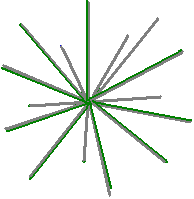The Strong force (short range) : 100 = 1 = Unit force
This is the force which powers the Sun, is the devastating force behind hydrogen bombs and the force that binds protons / neutrons into nuclei. This unit force is given the value of one, or ten to the power of zero (100), all other forces are measured as a relative strength of it.

Electro-magnetic force (long range): 10-2
This is the force that binds atoms and molecules together and is also the force of electricity. The source of power behind for instance a light bulb. It is one hundredth the strength of Unit force so, the value is 1/100 or one divided by ten to the power of two = 1/(102) = 10-2

Weak force (short range): 10-9
This is the force responsible for radioactive decay and instability in certain nuclei. It is one billionth strength of Unit force so, its value is 1/1,000,000,000 or one divided by ten to the power of nine = 1/(109) = 10-9

Gravitational force (long range): 10-38
This is the force that holds the Earth in orbit around the Sun, keeps our feet firmly anchored to the ground and makes apples fall from trees. It is 1/(1038) (one divided by one followed by 38 zeroes) 1/100,000,000,000,000,000,000,000,000,000,000,000,000 strength of Unit force so, the value is one divided by ten to the power of 38 = 1/(1038) = 10-38

The Gravitational force between any two objects for example the Earth and Moon can be measured using one of Newton's equations F = Gm1m2/r 2 . The constant G in this instance is the Universal gravitational constant. Meaning the force of gravity equals the product of any two masses divided by their radius (separation) squared, quantity times G.
The value of the Gravitational constant is 6.67259 E-11 ...m3 kg-1 s-2
The exact value for this is G = 1/(10 * √26 -1)c ...m3 kg-1 s-2
G = 1/(10 * √26 - 1)c = 6.6725903807971 E-11 m3 kg-1 s-2 Exactor
In this instance the speed of light "c" is just an amount of meters or a specific number , not meters per second.
Which gives us the very intriguing...perfectly repeating...continued fractional form ...
1/(10 * √26 - 1)c= 1 / (10 [5,10,10,10,10,10,10,10,10,10,10,...10 ]-1)c
that fits in really nice in our ten dimensional Universe.
Square root 26 = [5,10,10,10,10,10,10,10,10,10,10,...10 ]
that's 5 and 1/10 with another 1/10 tacked onto the ten in the denominators forever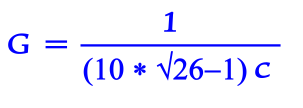```         1
5 + ----------------
1
10 + --------------
10 + 1/(10 + 1/(10 + 1/10... etc.))
```

#### G = 1/((10 * (√26))-1)c#### Flunot... center of axis (cut away view)

...So, this is the exact value for the Universal Gravitational Constant. But more importantly this defines the exact shape of the Unit Flux, it's a particle and by simply altering its shape it becomes anything and everything in this Universe. It has 10 hypotenuse or diagonals joined at their centers, terminating on the vertices of the dodecahedron.
This is the same as twenty radii emanating from a common center called a Flunot...

Arrangement of Axis Unit Flux 10-D
It has ten diagonals, nine with the force of light, one minus the charge or plus gravity (the normal state). They are composed of one dimensional (1-D) infinitesimal width string or tube like objects arranged in a ten dimensional Dodecahedron axes pattern terminating on vertices or the set of twenty points...```    (+-x/y,  +-xy,     0)  where y = (√5+1)/2
( +-xy,     0, +-x/y)        x = G /(20 * √3)
(    0, +-x/y,  +-xy)        G = 1/(10 * √26 - 1)c
(  +-x,   +-x,   +-x)
```
but, since we are at the quantum level where dimension is viable direction only, that makes ten dimensions in the actual space time continuum. The strings are only one dimension but are arranged in ten dimension Space...so the 10 different axes (20 different directions from origin) occupy or commandeer space in 10 dimensions without actually filling its volumetric area, thereby leaving it almost empty.
The Flux particles set up their own field (face centred cubic spherical space type pack) relative to the object of largest mass in proximity. This corresponds to a lattice which the center of every sphere or particle is the set of all points (x,y,z) where x, y, and z are integers adding up to an even number (That's arrangement, not size).

Flux particle...Nine Dimensional dispersion or 10-9One axis is used to convey the force...either photonic or gravitational, the other Nine axes are one dimension each.
This is a ten dimensional Universe so, every axis is a power of ten. When a force or vibration is being transmitted through one axis of any particle it causes the 18 remaining radii repel away from the charge. As the shape changes from nine Dimension (remaining axes of dodecahedron) weak down to two dimension (disc) electron it causes the particle to separate from the field connections lattice along the way.
The number of field connections is the determinate factor of relative strength or dimension of any particle. Any amount of repel or dimension change is possible here allowing force values from 10-9 through 10-2 on any particle or field created.

Electron...Can disperse energy in 2-dimensions or 10-2 strengthWhen one axis of any particle meets up with a proton charge and is held in place or a large force is being transmitted through it, two of the twenty radii are used as the Unit Force spike. The 18 remaining radii repel away from the charge into a two dimensional disc thus becoming the Electron.
This makes "18" the determinant number in Electron shell arrangements, check the larger noble gases: Argon, Krypton, Xenon, Radon, the amount of electrons in outermost shells will always sum to 18, the first three even have atomic numbers that are multiples of eighteen.
Three groups of six radii from one electron can form (along with seven other electrons) the corners of a cube or the "Octet Rule" (do the math...it works).

Proton...Zero Dimensions or Unit force strength...100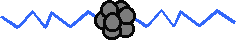With the positive or constricting force the 18 remaining radii curl into six types of Quarks in three flavors thus creating a proton with two string radii left. The flux strings always curl in groups of three (red, blue, green) with combinations of either charge (frequency) or rest (zero) giving the Quark a characteristic One third or Two thirds spin flavor. A group of two is the Proton - Neutron - Connector Meson.
If the proton were the size of the dot above the letter "i" then the strings could be compared to something a lot finer than the web of a spider extending out a few hundred meters, fine enough where eighteen strings can curl into a space the size of the proton and have a spaghetti ball type configuration with a very loose string (or filament) pack.
It is the way to make the most universe with the least amount of material.
Newton's equation F = Gm1m2/r 2 breaks down and becomes ineffective at the proton - electron size or atomic realm, the particle shape has altered and the actual mechanism of attraction or attachment has changed.

Neutron...Nineteen curled radii, one spike left
(dying to curl up with a proton spike)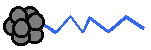The Neutron has one more string radius curl than the proton giving it slightly more mass. Protons can chain together as they curl, a Neutron is a nice way to dead-end the chain so the force of Gravity can ensue.
The string radii or spikes are the mechanism any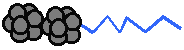particle...electron, proton or neutron etc. uses to connect and relay charge or vibration. In the atomic nucleus the last spike (one radius) of a neutron and one of the two proton spikes curl into a meson, that leaves the whole proton-neutron package with only one spike emanating from the proton, thereby leaving the neutron without an outlet, giving it a neutral charge.
This means an actual free neutron will have all twenty radii curled. If one curled neutron radius suddenly uncoils... it will convert into a proton with the ejecting charge or vibration creating an Electron in the process and is called Beta Radiation (one diameters worth of charge is an Electron or in this case one half... spin?), note: if the curled radius in the proton / neutron connector opposite the proton spike actually breaks off from the proton, once again the neutron radius suddenly uncoils creating the electron and proton but this time there is a free radius or diameter also and is called a neutrino.

Quantum Weirdness:
Electrical energy is used to power mechanical devices but at the quantum level Electrical energy is just a frictionless mechanical vibration.

 Properties of Energy & Matter * part III *

 by James Clifford Cranwell 4/01/98 Part III ... Gravity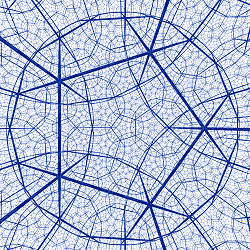As Star light travels through the Universe, what actually happens is a specific frequency or vibration (a photon) travels along one or more of the flux particle axes strings. The flux particles are arranged in a Field or matrix.
The matrix is held in place by the gravitational effect (the attraction of particles to each other) and curved around any mass in proximity. Light energy or electro-magnetic expansive force adds to the rigidity of the field matrix, but no matter how great the strength of any individual segment of a lattice structure, it is easily folded up or warped by any other force, in this case Gravity.

The DodecahedronThe Dodecahedron (DDH) is the pattern or axial arrangement used by the unit Flux, it is stackable hyperbolically and absolutely the most efficient way to pack space with the least amount of material (smallest angle of symmetrical invariance, any more than ten axes per particle and they won't connect).
The only problem is the vertices are tri-linear (where any axis terminates it will intersect with three others). If a frequency traveling along an axis passes directly through the center, then out any of the tri-corners, that same linear direction will not pass through any other of the twelve Dodecahedrons in proximity.It will be directly on the edge where any four Dodecahedrons meet. So the individual Photon which we now know is one vibration traveling along the Unit Flux strings can never take a straight path. The vertices where the Dodecahedrons meet have four paths.
If you place three base balls on the ground in a triangle then place another on top to make a pyramid shape, that will be the way the base balls and Dodecahedrons stack. The only problem here is where the base balls touch each other is not where the Unit Flux Strings are located. The end points the Flux Strings terminate on tri-corners. Thus sending the intrepid photon on a Zigzag, Corkscrew, and other intricate paths destined to scatter.
It is easy to angle out of position with nothing else around. It is the combined effect of many photons initially heading in one direction with momentum that keep each other in line and in proper direction except of course near the scattering outer fringes. This is the reason why one photon particle traveling in a zigzag can also be interpreted as wave function. Its path is forced into a wave type motion. A vibration sets off resonant vibrations in itself, perpendicular to the original path. Thus creating other fields and background radiation.

Graviton...38 Dimensions or 10-38 strength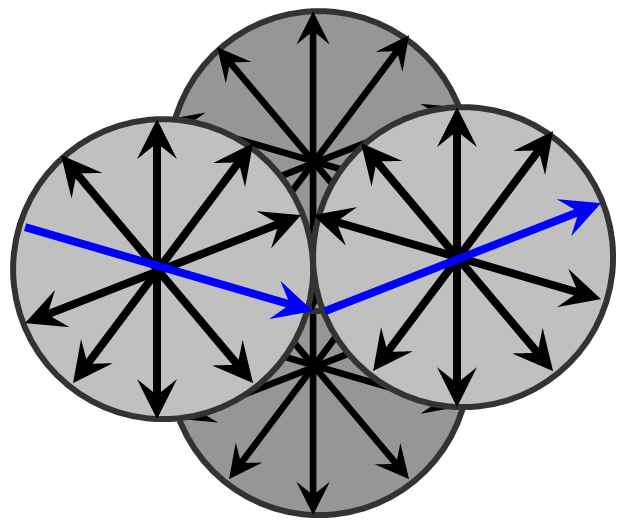Drawn here with spherical encasement for clarity, this is the way Flux pack space and are compressed in proximity to a large mass. To complete one wave of pull the gravitational attractor charge takes on its journey, one complete wave of the zigzag must be used to stay in any given direction (remember the Flux axes intersect in groups of four).
So for this package that's nine dimensional Flux dispersal times four unit Flux or 10-36 strength, if only two paths are used through the group that makes the dimension of the group 10-38 or Gravity. If we replace the G in Newton's equation F = Gm1m2 / r 2 with the exact value we get F = m1m2 / ((10 √26-1)c)r 2 then we can easily see the masses divided by the radius squared times the length in question equals the force of gravity.

No problem curving light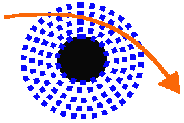The flux particle field is held in place and curved around any mass in proximity and pulled in and compressed thanks to the lattice type arrangement of the vertices. If the gravitational force is strong enough (an infamous (supposed) Black Hole made entirely of protons or neutrons... same thing et.al.) the Flux will flatten down to an electron like 2-D shape with the normal or volt spike included in the fold, giving the surface structure of the Black Hole the same functionality and appearance as a giant Electron in space.
Once again Newton's equation F = Gm1m2 / r 2 breaks down and becomes ineffective, the whole particle field shape and dimension has changed. The overall composition could be considered a gigantic atom in space. The Flux are completely compressed at the surface but make a smooth interdimensional transition back to a point in normal space. This means not only will light escape or discharge but it will be an immensely brighter light than is possible as the result of any Stars Hydrogen fusion reaction.
As you approach a Black Hole there will be a dramatic increase in gravity. The zigzag (lattice structure) of particle matrix axes increases, folding up like an accordion. The distance light has to travel increases thereby slowing it, compressing time and space along with it (from an unaffected observers reference frame). But it will take longer and longer to pass through the compressing field so, as the field compresses the overall effect is time will slow down and you will compress along with the field... you might not even notice (until it's too late), you will not stretch out.
(special note: Gravity is the attraction of particles pulling complete groups of atoms together. In a black hole there is a continuous proton / neutron pack and they have shedded their electrons, and although the protons / neutrons are held together with the strong force, i.e. one radius each curled up together, there is nothing to actually compress them together as a group. It would be something like a pearl necklace, any pearl might be strongly connected to its neighbor, but if you folded them up into a ball, there is nothing to collapse them together into a supposed singularity, they are connected but they are not pulling. )

Cosmological Constant: Fifth Force : Dark Energy : Quintessence : Æther : The Aether

The speed of waves of any system is given by the equation: Velocity "v" of propagation equals the square root of the quantity Tension "F" divided by the Mass "u" per unit length ... v = √( F / u ), squaring both sides we have: v 2 = F / u .
In our section of the universe, we know the velocity is the speed of light, so let's assume c2 = F / u . Even though the values for tension and mass are unknown, it's reasonable to assume the mass of space is vanishingly small, that makes the tension of the field tremendously greater than its mass (the ratio is the speed of light squared: 89875517873681764).

When any flux particle is in free space it has vibration or light energy from billions and billions of stars traversing it in every possible direction. Although every particle is attracted to every other particle, without any large force or vibration on one specific axis, every axis will repel or expand away from every other axis, attempting to expand the whole field to its greatest volume. This process can be considered a universal expansive force, the Fifth Force or Dark Energy.

An easy to understand two dimensional model of the Flux Particle Field would be something like a highly stretched tennis net made of the finest spiders web, and in this model "matter" would be a balled up piece of the web stuck to the net (everything is infinitesimally small). This field is not stationary in space with other things rushing through it like MM mistakenly assumed. It is everywhere and surrounds matter. (note: it might be stationary way out in deep space where there are no planetary, solar and galactic, etc. Movements)

It works in the same type of manner as gravity (a group effort to push or pull in any particular direction) but opposite in force direction. Every particle attracts every other particle but that just creates the field. Nothing can actually happen until an irregularity is introduced into the system, for instance any particle becoming confined as proton mass. Then the field will curve around it and it will be drawn to and/or attract anything in its realm. Gravity and force five ratio varies at different places, for instance near a star there is gravity and a tremendous light energy or expansive force, but on Earth there is gravity and only residual sunlight (expansive force). Gravity ( the actual particle attractions) are the major factor in drawing in a mass and curving the field. The centripetal force along with expansion keep it at bay. Remember... no matter how strong an individual segment of lattice structure, the whole structure is easily curved or warped by any other force. (if something is packing itself around something curved, like the surface of any planet or star, it will... you guessed it, curve.)
Yes, all these things...
Cosmological Constant: Fifth Force : Dark Energy : Quintessence
... are what others have been unknowingly calling the the stretching property of Flux Particle Field.
The field itself (and any loose particles) which completely fill space is unwittingly called Dark Matter: Æther : The Aether etc.
One indivdual particle has been inadvertently called The God particle. etc.

gravitational warpage :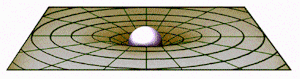The stretched rubber bed sheet and the bowling ball aren't an accurate model of the gravitational warpage of space... that implies the area nearer the sunken ball is higher density and other masses are attracted to it.
The reverse happens, particles seek a lower density, as in (something like) pressure. You can't say the higher mass or density has a higher gravity because you are using gravity in the example (the explanation) and you can't because in this case it's the question. In this model "gravity" is causing the acceleration downward not the curve. If you stick this model in space and incline the plain there is no effect. Space (everything) must have a actual field like explained. (Note: my 2-d and 3-d are different from what we're talking about here)
...Also, if gravity (the actual pulling effect) is caused by a warping of the supposed void of space, there would be a measurable difference in even a miniscule range, for instance the opposite sides of a piece of paper held horizontally. If we stick this whole scenario in a vacuum, the paper would drop like a lead weight, leading us to believe there is an impossible different warp or curve per micro-meter. The force of gravity at the surface of the earth (for instance) and lets say 300 yards up, are about the same, correct? But now if you think of the sheet of paper in a vacuum... there is enough difference even in the range of a micrometer to make it drop like a lead weight, ...there is so much difference/change on the opposite sides of paper that it drops like lead? Does that seem right? Do you see my point?
In a micrometer there is a dramatic difference but 300 yards is the same? No, somethings really wrong with that thinking, it's backwards.
Just about the same "supposed" curve at 300 yards would be negligible at the micrometer, what's causing the pull?
The picture itself bothers me also... it uses a 2-D model (the sheet) to represent something that can't be represented without sticking a 3-D bowling ball in it and including "gravity" (rolling downhill) without an explanation. And space itself is supposed to be one dimension higher than mass, not less. If you wanted to bump everything down from what is perceived as actuality to those dimensions...
you would have to take a...
3-D earth and 4-D space and reduce them to a...
2-D earth and 3-D space, not the 2-D space (sheet) like the diagram.
Four dimensional space is curved? Where or what is the curve? Is it thicker closer to the source of gravity? Why would something be attracted to a thicker area/density?
Gravity isn't curving space, it's (space is) curved because the Sun or a planet is spherical. If the Sun were an infinitely sized flat object there wouldn't be a curved field but it's safe to assume there would still be what we call Gravity.

---------

 Properties of Energy & Matter * part IV *

 by James Clifford Cranwell 3/12-14/98 Part IV ... Atomic Structures

Charmed
It's ludicrous to believe there was a Big-Bang, then billions and billions and billions etc. of groups of different type particles ( Muon, Alpha, Down, Electron, Lambda, Yada, Neutrino, Green, Blue, Eta, Red, Gluon, Tau, Pion, Kaon, Proton, Neutron, Sigma, Xi, Omega, Up, Strange, Photon, Charmed, Bottom, Top, Positron, Meson, Baryon, etc.) all magically formed themselves together into exact arrangements to become elements. How do they know how to arrange themselves without any external guidance?
This implies two things...
No.1 morphic field : There is some kind of morphic field guidance system that arranges particles into matter as we know it, exact same structures every time. If this is so, then not only are there all these types of particles, there is also another field (morphic) to describe. The only difference here is the morphic field isn't the effect created by electron movement and charge, it's a field that controls everything, it's the cause. That implies the morphic field has a brain or some other type of intelligence controlling it (perhaps yet another morphic field), that is quite impossible.
No.2 pre-formed matter : All particles and atoms just happened to be already formed. Hydrogen, Iodine, Magnesium, Sulfur, Gold, Oxygen, Nitrogen, Silver, Krypton, Iron... you name it, it was already formed. If that's the case, how did everything get formed? Was there a Morphic field? Were the heavier elements created in the hydrogen fusion of stars? Where did that hydrogen come from? Was there a Morphic Field?

Cause and effect:For any action to take place there must be an underlying cause, something where the end result is the action in question, therefore we have to realize there must be something causing the cause, and something else causing the causing (the middle-man cause). This will tend toward infinity with no explanation of an initial cause unless of course there is something that behaves automatically in an exact manner when an outside influence affects it, an Automaton. If there is a vibration on it, it will expand or repel and convey the vibration (if something is vibrating it automatically shakes everything in proximity away).
If there is a lack of vibration or state of rest it will curl up and pull. Now the whole thing is simple, at the quantum level or the actual beginning of particle interactions things have to be this simple, anything else implies and endless string of cause and effects. Thinking there is more than one type of particle in this Universe is ridiculous... if there is more than one type of particle and there isn't an infinite number of particles, then there is an absolute number of different type particles, for instance twenty-nine or thirty-seven.
How are all the different type particles magically finding each other in order to be formed into matter? Do they just happen to be in the right place at the right time billions and billions of times (for even the head of a pin) everywhere in the Universe? Is there a Morphic Field Cousin that moves matter from place to place and knows exactly how much matter to move and where to move it? What's controlling this Morphic Field Cousin, a Morphic Field Uncle? The only questions here are... Why are there particles? What caused the initial vibrations or lack there of ?

Basic Particle Construction:
They're right in front of our face, we're made out of them, ...Everything is made out of them. So, where did the basic flux particles come from and how were they formed? Although their ultimate creation or coming into existence is unexplainable there had to be some form of matter or substance from which everything was initially formed or else we wouldn't be here. And the most efficient type of construct is the string,...if it's just a coincidence, it's the most amazing coincidence that ever happened.
So... if we have a bunch of strings floating around how do they automatically form into the ten dimensional or twenty radii from center shape? That's easy... if any strings join together at their ends and then more strings are attracted to the joint... it will build into the shape in question. When it reaches a point where there are twenty strings sticking into one center, that's the point where no more strings will fit all the way into the center of the package, it's the cut off point. It's the vertices of the dodecahedron or the faces of the icosahedron ...the flunot... center of axis. The flunot will automatically form then everything else will automatically form from it... it couldn't have happened any other way.

By the way:
What are all those different particles their talking about? Their just a certain amount of the string particles from a busted up proton, that's why there's a distinct ratio between all the supposed different particles MeV, something might be three strings broken off but still balled up together something else might be nine strings still balled up.

Sub-Quanta:At the quantum level there isn't anything we can actually perceive as color or shape. Color is a specific frequency (a photon) traveling along one or more of the Flux strings. All we are able to see are the vibrations in a direction toward us... not the string or particle itself.
... Analogy: ...we can use a voltmeter or a galvanometer to measure current or voltage through a wire, but there is no chance of the voltmeter or galvanometer seeing (actually looking at) the wire. An human brain, like the galvanometer, is just a measuring device and it is only measuring frequencies that we perceive as color, we can't see the wire (flux strings).
The electron radii are the spines of an umbrella like structure without the fabric (The fabric of space doesn't have fabric). Light or vibration is conveyed to the atom then is added to the existing charge or vibration quantity until the threshold is exceeded and a quantum leap is made by adding other flux as connectors between electrons. This will give an instantaneous change in orbit or shell size.
This process also changes the amount of repel or what we now know is actual "dimension" of individual particles in specific amounts. They can load or vibrate up to full 2-D charge, then upon discharge will be released in discrete packages or amounts and are called photons. Electrons travel through free space at the near the speed of light. The speed of light is a vibration along the Flux particle field.
An Electron force is an actual shape conversion of the particles as it traverses (a pulse like snapping in and out of 2-dimensions ). That's more work, so, it takes slightly longer. A stronger Electron force, for instance lightning, also has the effect of straightening out the zigzag property of the field lattice, thereby totally disrupting the proximity space-time.

Quantum Mechanical:
... A force is something that causes action to take place, and although there can be any number of forces working in unison or opposition. There can also be a series, or one force that is a direct result of another force, "this caused that". But, there is a limit to the number of forces that can be working in series, because there has to be an initial force or an ultimate beginning of any series. This can be quite perplexing, if we are at the ultimate beginning in the force series chain, there must be something causing the initial force, and it can't be another force or else we are not at the beginning and there will never be an initial force or beginning. So the initial force can't be a force at all. It can only be an intrinsic Mechanical vibration.

Quantum Weirdness: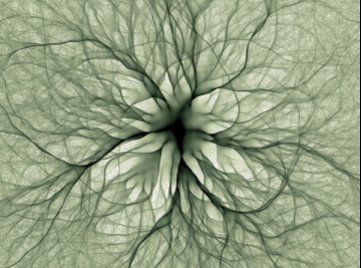... At a size or realm smaller than the electron orbital radius itself, light doesn't enter (light is only a vibration) . Only highly charged particles with velocity high enough to disconnect from the continuum can. Any scientific measurements made in this manner are actually accomplished outside of space-time.
Any particle that has charge high enough to disconnect from the field will have a speed completely irrelevant to light or anything else. A particle is actually something, a photon is only a vibration on the particle...
if you hold a piece of rope, you have a rope... if you shake the rope, you have photons... does the shake or vibration have mass? If we use this as an example of an atom and magnify the nuclei (proton) until its size is proportional to the dot above the letter "i" ... the ropes or strings would be like one silk strand of a spiders web (per proton) extending out a few hundred meters to the electron, it (the strand) would have to be fine/thin enough where eighteen or nineteen of them can curl up together into a spherical space the size of the proton, because they (the strands) actually are the proton.
The speed one wave crest vibration travels along the string would be considered the speed of light... any balled up string zipping by has little or no chance (31,415,926,535 to one)* of coming in contact with anything and completely irrelevant speed and existence.

* 31,415,926,535 to one... that's the area of one slice taken through the atom with the nucleus being the bull's eye and the slice area equal to pi radius squared or pi 100,000 2 ...

 Properties of Energy & Matter * part V *

 by James Clifford Cranwell 2/01/98 Part V ... Relativity
If there were only two objects in the universe (hypothetical), an observer and the Earth, both completely weightless / massless , the observer would be tricked into thinking he / she has weight because of the gravitational tug and mass because of the large concentration of gravitational tug, in their reality there are only forces. The Mass of the actual Flux particle is negligible... it is substantial only when it has a vibration or a gravitational pull on it. Therefore Mass is only a vibration or a pull...it isn't something we can actually take to the beach. Proof...

Note: some of these examples are intended only to show a direct correlation or relationship between everything ...inclusive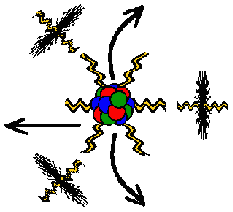E = mc2 (Energy equals Mass times the speed of light squared) and...
E = hf (Energy equals frequency, h is constant).
Therefore, we can replace E in the first equation with hf and get the result hf = mc 2
When Mass is released as Energy it leaves the nucleus as Electrons traveling through free space at the speed of light...don't forget...the Electron is two dimensions so, Energy equals Mass traveling at the speed (or length) of light in two dimensions or E = mc2
This can be thought of as the balled up strings of protons and neutrons (the mass) contained in the nucleus suddenly expanding, actually creating more of (what we call) space and vibrating away from center as 2-D electrons

A quick textbook type dimensional analysis gives even further insight...
The E or energy in this case is measured in joules with dimensions of [M][L2] / [T2]
That's mass times length squared divided by time squared
The m part of the mc2 is mass with dimensions of [M]
So far we have the energy part [M][L2] / [T2] = and the mass part [M]
To make the right side equal the left we have to multiply the mass by [L2] / [T2]
that's length2 divided by time2 or meters2/seconds2
Speed of light "c" is measured in meters per seconds [L] / [T]
Squaring this we arrive at the needed [L2] / [T2]
Is there anything that has the dimensional form [L2] / [T2] already?
What did the gravitational constant look like?.......m3 kg-1 s-2 = [L3] / [M][T2] units

In an atom, most of the Mass is contained in the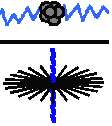nucleus. However, an Electron does have mass and the Proton / Electron mass ratio is 1836.1527556561 , As a result of this division we have canceled the strong force of light / gravity on four radii of the Electron-Proton combination in this example and we are left with 18 inanimate or inert radii of the Electron shell and the 18 curled radii of the Proton. Now we divide 1836.1527556561 by 10 square root26 (the exact value without the cancelled speed of light and gravity portion...negative one times speed of light, these were cancelled in the initial division) then divide by 36 (the number of curled radii and inert radii) and get the difference 1.0002755054517 ...This means everyone's measurements are correct to within two / ten thousandths and the ratio difference between 36 times 10 square root 26 and 1836.1527556561 is almost unity.

E = mc2 :
Energy equals Mass, speed of light squared is constant. As a result of the fusion reaction in the Sun, a tremendous amount of mass is converted to energy. Therefore, space is being filled with mass that has been converted or changed into energy. Since mass can be called particles and energy is the same as mass only in a different shape or state, we can say that space is filled with energy or particles, it isn't a void.
E = hf :
Energy equals frequency, h is constant. If we consider any point in space, we realize it has frequency or energy traversing it from every direction, this same process is happening at every point in space. Therefore every point in space contains energy or mass. Therefore if the Earth can be considered to have mass, space has an actual mass and cannot be a void.
E = hf = E = mc2 :
hf and mc2 are both equal to E (energy),
this means hf and mc2 are equal to each other ... hf = mc2.
h (plank) and c2 (Speed of light2) are dropable (ignorable) constants so,
f = m (frequency equals mass), therefore frequency must have conveyance or a particle involved in its travels or else there will be nothing to contain energy when it converts back into mass. It works almost the same as electricity in wire. Particles are released from their mass or rest states and fill space at the speed of the solar wind. It is only the vibration effect conveyed on the particles that travels at the speed of light.
Quantum Weirdness:
The speed of light is only an effect created in the particle field, there shouldn't be any problem traveling faster than it, except... every type of propulsion now uses something related to the same electron field vibration speed, for instance, chemical changes, nuclear reactions or even some type of electrical/magnetic propulsion would still be using the same electron vibrational speed, how can you travel/go faster than something that you have to use to go?
If you could travel fast enough to break free from field interactions you may find the speed of light incredibly slow (although there will be no way to discern this). A possible problem... once you have broken free from field interactions and are out of the space time continuum there is an absolute lack of reference frame and you become neutrino like with a speed and existence completely irrelevant to anything known. It may be impossible to re-enter or slow back into this space time.

 Properties of Energy & Matter * part VI *

 by James Clifford Cranwell 4/19/98 Part VI ... The Big Bang Myth

Energy equals frequency ( E = hf )
Area Increases, Energy must divide.Light energy wave vibrations expand spherically away from their point of origin (The Star), also the waves or vibrations are expanding away from each other. There is more and more area to cover with the same unit amount of Energy.

What this means is light vibrations along the strings propagating through space will be divided to fill the ever increasing area. This is accomplished by a reduction in frequency or an increase in wavelength and is the reason for what is otherwise known as the Red shift (except of course some actual random movement). The further away, the greater the division or separation of particle vectors, the more red shift.

The Universe isn't expanding, although the fusion reaction in the sun and other stars does allow the release and expansion of billions and billions of Flux particles from confinement in nuclei back into their normal state, this excess amount will generate raw material for the formation of other matter elsewhere in the Universe and give space an ever changing star field by a type of cosmic convection. Stars radiate spherically so, their energy, matter or light is distributed evenly throughout the cosmos... and since the light from all stars also converges or can be seen from any point in the Universe this means we are a small part of everything in the Universe.
The Universe extends to an inconceivable infinity. However far you go into Space there's more Space; filled with Planets, Stars, and Galaxies with no place left to expand into... Think about it... the Universe can't expand... it's already there.

Big-Bang...not this time.

There wasn't a Big Bang, everything can't be assembled neatly in a package somewhere, you can't do that with infinity. The Universe can't collapse under its own forces, everything is being pulled on from every direction (no matter how far a small mind thinks the edge of the universe is, there is still an infinity beyond). Nothing can assemble to a point where it contains everything.

Thinking there was an original point of inception (Big Bang) and everything is racing away from it is the same as thinking the Earth is the center of the Universe and everything else revolves around it.

Different distances will have different Red-Shift value

Wavelength will increase at differing rates at different distances... greater initial shifts then less as sphere of light in any given direction increases (for all intents and purposes) to almost parallel. If Star S401 and Star G401 are absolutely motionless in relation to each other and the observer they will appear to have a different red-shift if they are at different distances. Our Sun is included although it can't be regarded as a point source like the more distant stellar counterparts.

Actual movement clouds the issue even further.

Actual movement of Star relative to the observer will cause increase or decrease in amount of Red-Shift over a given time period. If Star R327 actually is racing away and the red shift is noted, then some time later its red shift is measured again and it has increased, it is not racing away faster... it's racing away constant. Only the amount of energy its light has lost because of position has changed. Blue shifted starlight from an incoming Star can actually be red-shifted back to zero shift and falsely appear to have no movement.

Other possible reasons:

• After traveling billions of miles and years, light just becomes tired and slows down.
• The suns fusion reaction is constantly increasing the mass of space by dumping more and more of what we actually call space (flux) into space thereby increasing its mass... Velocity of propagation equals the square root of the quantity Tension divided by the Mass per unit length ... v = √( F / u ) if the mass increases the speed goes down.
But this would have the opposite effect over billions of light years. If the density of space at two points is the same (lets say low). Then the density steadily increases over time, when we look back in time through a telescope we would still see the low mass space with fast moving blue-shift light. So Maybe Black holes are absorbing more space than is being emitted by stars thereby lowering the density over time, then when we look into deep space billions of light years ago we would see high density space with slow (red-shift) light.

The point here is there can be perceived shift without actual movement.

Quantum Weirdness:

We are here... therefore an infinite Universe. There cannot be an edge or any type of end to the Universe. Any wall will always have something on the other side.

 Properties of Energy & Matter * part VII *

 by James Clifford Cranwell 10/06/98 Part VII ... Miscellaneous
parallel dimension :
We can only see things in our continuum, vibrations in a direction toward us along the strings. The nucleus radius to electron orbital volumetric empty space ratio is onequadrillionth ( 1 / 1,000,000,000,000,000 ). Since the flux in free space are not curled into supposed nuclear mass, they can assume or commandeer more free space volume... there's not even a proton radius to contend with now. That makes the free space in the Flux particle field greater than 99.9999999999999% ... there can be an almost infinite amount of material in the free space between the strings (infinity minus the one quadrillionth infinity field). So anything small enough (a Neutrino)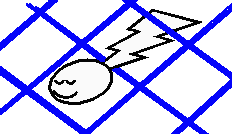can be in or out of our space time continuum thereby having a speed completely irrelevant to that of light and an existence completely irrelevant to ours (...easy to see how non-local phenomena can happen).
The lattice structure of the fields also allows for one or more proximity fields without any intersection. This means mass can have parallel fields surrounding it and occupying the same space, creating an higher density. Anything that absorbs or reflects light (vibrations) or disrupts the field in some manner will have a discernible shape, anything between the strings is invisible.
There can be an independent field, A micro-swiss-cheese type mass or a fluidic type matter unconnected to the continuum, unaffected by light, gravity or matter... completely invisible to us, unless there is an instantaneous change in Flux field dimension thereby rendering it momentarily visible, if (and only if) it can somehow actually connect and if it is even possible for there to be more than one type of matter.
Question: can one or more axis or radii break away from the Flux, curl up, and become something? What would we call them?

Superconductor :
If you study anything, you change it. If you measure an electron, for instance, you will change the state of the electron by absorbing some / all of the electron energy vibrations into the measuring device. So, although it may be possible to create a frozen torus or donut shaped object with an almost perpetual electron current flowing through it, once the field created by said object is tapped for its current inducing magnetic field properties the source will simply drain or transfer like any other source of energy (it's our old friend... cause and effect), you have to pay for your lunch.

Time travel :
Nope.

Dark Matter :
What they call "Dark Matter" is simply the field of flux particles completely filling space (explained in this theory).
Can you see the field or particles? No, it would be like trying to "hear air", you don't hear air, you hear vibrations happening in the air. You can stick this whole scenario under water and it's the same... you hear the sound of for instance a whale but you don't hear the actual molecules of water, you hear the vibrations in the water.
You can't see a particle, you only see vibrations from the particles.
Once again... if you hold a piece of rope, you have a rope... if you shake the rope, you have photons... If the rope is shaking at an high frequency, your eye will detect one pinpoint spot of blue color (from the end of the rope). If it's a slower frequency your eye will pick up a red color. You never actually see the rope.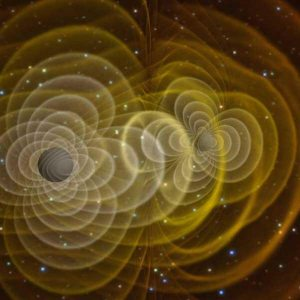Michelson-Morley Experiment :
Michelson-Morley Experiment won't work if the field is moving along with the experiment apparatus, it's actually ridiculous, they thought it out pretty good but forgot one thing and it became garbage.
Can any of you ding-dongs understand that? If you are on Earth, and there is a field directly connected to the earth. You can set up all kinds of mirrors and other things and twist and turn them and it just won't matter because the field is moving with the earth.

Molecular combiner :
When there is a lack of vibration Flux particles are destined to curl and be confined as mass, To break them free from this restive state and set them in motion once again will take energy, so to create matter you have to take away energy. This means a matter making machine or replicator will create a surplus of energy, not only from a proton and neutron combining one charged radius each and ejecting an electrons worth, also from whatever residual quantum fluctuations are left on the free flux particle before it is combined into a proton.
Note: there are two completely separate and different ways to look at this (of course).
One is combining atoms and molecules already in existence into different structures. The Other is somehow converting the actual field or particle strings into Protons and neutrons. If you have elements you can make anything, the question is how do you make Hydrogen out of supposed empty space.

Casimir Effect:
Zero Point Energy (ZPE), or vacuum fluctuation energy are terms used to describe the random electromagnetic oscillations that are left in a vacuum after all other energy has been removed. If you remove all the energy from a space, take out all the matter, all the heat, all the light... everything, you will find that there is still some energy left... The Flux, you can't remove the flux, for it will always connect to any apparatus of evacuation thereby reviving itself. Any apparatus of evacuation or metal plates are made of atoms with orbiting electrons with charge.

Entropy :
Energy is just a mechanical vibration, these vibrations happen in this universe, they have no where else to go. They can divide into lower and lower frequencies, add together and change states but never dissipate into oblivion. It's the cause and effect scenario once again, there isn't anyway to stop a vibration because the stopper will be affected, thereby continuing the process.
If there were an hypothetical edge to the flux particle field (hypothetical edge of the universe), vibrations would travel to the edge of the field, run out of particles, have no where to go and be completely reflected (180 degree phase change) back and almost focused, the same effect as a spherical mirror (assuming the edge is curved), thus creating the same effect as deep space telescope, reflecting the light of possible nearby objects (observer to object) from a very distant past (object to edge of universe then all the way back to observer). Any pocket of space that is void of particles or any break in the field where vibrations cannot traverse has the same effect. Therefore energy remains.

The Limit :
Mathematical equations are a great way to describe the physical conditions and properties of Matter except when an infinity or a zero denominator is thrown into the equation, then it might not actually work in the real world. In the same way Zero Kelvin (negative 273.15 Celsius) is an absolute zero or a temperature limit and calculating molecular movements mathematically at for instance, negative 4001 Celsius would be ridiculous. The amount anything can compress to or exert force on also has a limit. You can write anything you want on paper but, mass isn't going to collapse to a pinpoint and thereby make the gravity approach infinity.

Raisin bread :
The Raisin bread model of expansion is also a farce, it can't expand because if the universe were a loaf, it would have to be an infinite sized loaf, or an infinite amount of loaves with no room left to expand.

 Properties of Energy & Matter * constants *

Home Page     cranwell@gootar.com     https://xulfrepus.neocities.org/